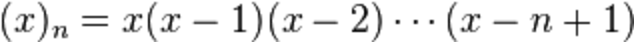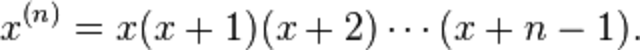# Re: Factor

Factor: the language, the theory, and the practice.

## Factorial

Monday, April 15, 2013

Calculating factorial numbers is frequently used to compare programming languages. Usually the implementation is simple, as is the one in Factor:

``````MEMO: factorial ( n -- n! )
dup 1 > [ [1..b] product ] [ drop 1 ] if ;
``````

I hadn’t realized until I skimmed the Wikipedia article on Factorials that there are actually many more kinds of factorials and what Factor really needed was implementations of all of them!

### Lots of Factorials

primorial

Similar to the factorial, the primorial is the product of the first n prime numbers.

double-factorial

The product of all the odd integers up to some odd positive integer n is called the double factorial of n, and denoted by n!!.

multifactorial

The multifactorial is a product of integers in steps of two (n!!, the “double factorial”), three (n!!!), or more (in general for a given k step: n!(k)).

The so-called quadruple factorial, however, is not the multifactorial n!(4); it is a much larger number given by (2n)!/n!.

super-factorial

The super factorial is the product of the first n factorials.

hyper-factorial

The hyper factorial is defined as:alternating-factorial

The alternating factorial is the absolute value of the alternating sum of the first n factorials.exponential-factorial

The exponential factorial is a positive integer n raised to the power of n−1, which in turn is raised to the power of n − 2, and so on and so forth.

Be careful with n > 4: the exponential factorial of 5 is 5262144 which is approximately 6.206069878660874 × 10183230.

factorial-prime?

A factorial prime is a prime number that is one less or one more than a factorial.

primorial-prime?

A primorial prime is a prime number that is one less or one more than a primorial.

falling-factorial

The “descending factorial”, “falling sequential product”, or “lower factorial”.factorial-power

A generalized version of falling factorial.

rising-factorial

The “ascending factorial”, “rising sequential product”, or “upper factorial”.### Now available!

That’s probably more factorials than anyone really cares to know about, but now Factor has more than ten times as many factorials as before! The code could probably use some cleanup, but it is available now in the math.factorials vocabulary.